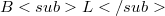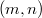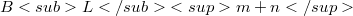﻿

### Existence and uniqueness theorem for Frenet frame supercurves

#### Abstract

In the first part of this paper,using the Banach Grassmann algebra$BL$ given by Rogers in her paper ,a new scalar product and a new definition of the orthogonality are introduced on the$(m,n)$-dimensional total supereuclidean space${BL}m+n$. Using the GH∈fty functions given by Rogers in , the new definitions of the supercurve, of the supersmooth supercurve, of the supersmooth supercurve in general position and of the Frenet frame associated to a supersmooth supercurve in general position are given. In second part of this paper, using the classical results described in , the new existence and uniqueness theorem for some supercurves which admit Frenet frame is proved.

DOI Code: 10.1285/i15900932v24n1p143

Keywords: \$(m, n)\$-dimensional total supereuclidean space \${BL}m+n\$; The \$(m, n)\$-dimensional supereuclidean space \${BL}m+n\$; The \$GH\$ functions; Supersmooth supercurve; Supersmooth supercurve in general position; Frenet frame associated to a supersmooth supercurve; Frenet formulas for the supersmooth supercurve

Classification: 58A50

Full Text: PDF